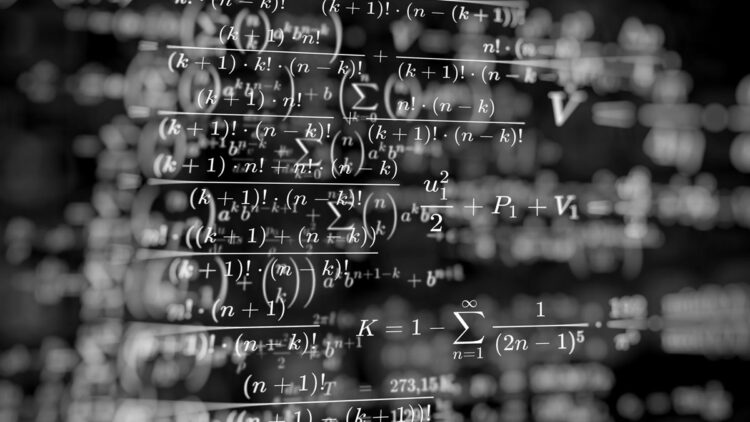# 7 Hardest Math Problems In The World (Unsolved)

By Duane BeckettComplicated math is intense and detailed. And advanced math problems are undeniably the hardest math problems. Many of which remain unsolved.

These are the toughest in the world:

## 7. The Large Cardinal Project

German mathematician Georg Cantor had figured out that infinity can come in different sizes, and some infinite sets have more elements than others:

Mathematicians keep discovering larger and larger numbers or sizes, known as Large Cardinals. So the process goes that a definition for a cardinal is arrived at until someone proves that another cardinal is bigger than all other known cardinals. Then, depending on their proof, that becomes the new largest cardinal.

Throughout the last century, the known large cardinals have steadily been pushed forward. While the top of the large cardinal hierarchy appears to be in sight, many questions remain open about how big this final large cardinal number will be.

CHECK OUT: 10 Unexplained Ancient Artifacts From Around The World

## 6. Unknotting Problem

Today, the simplest version of the Unknotting Problem has been solved, but the full version remains unsolved. The basis of this problem emerges from the math topic Knot Theory:

The existing algorithm can unknot the knots of any complexity, but when the knots start getting more complicated, the algorithms start to take an impossibly long time to solve.

So what remains to be seen is if someone can develop an algorithm that can unknot any number of knots in what is known as polynomial time, this will finally solve the Unknotting Problem once and for all.

(However, at the same time, someone could even prove that this problem is unsolvable).

## 5. Kissing Number Problem

In the top five of hardest math problems is the Kissing Number Problem:

The Kissing Number Problem specifies the following – when there are numerous spheres packed in an area, each sphere is said to have a kissing number, which is the number of other how many spheres it is touching.

For example, if you are touching six neighboring spheres, then the kissing number is six.

A packed bunch of spheres will have an average kissing number to help describe the situation in terms of mathematics. However, it is when the problem crosses three dimensions or large numbers that the Kissing Problems remain unsolved.

CHECK OUT: The 7 Most Valuable Foreign Coins: From Europe To Africa

## 4. Riemann Hypothesis

One of the most important open problems in math is the Riemann Hypothesis. There is even a million-dollar reward for its solution:

(That’s right, this is one of those million-dollar math problems you’ve no doubt heard about).

The Riemann Hypothesis focuses on all non-trivial zeros of the Riemann zeta function is in real part 1/2.

In terms of complex numbers, this means that the function has a specific behavior along a vertical line, and the hypothesis states that this behavior will continue along this line infinitely.

The understanding of prime numbers has developed significantly in the last 160 years, but there has been no solution to the Riemann Hypothesis yet.

## 3. Twin Prime Conjecture

The Twin Prime Conjecture is one of the most famous unsolved problems in math:

It is one of the many number theory problems, involving prime numbers. When two primes have a difference of two, they are called twin primes.

For example, 11 and 13 are twin primes, as are 599 and 601.

Number Theory states that there are infinite prime numbers, so it should hold true that there are infinitely many twin primes. Accordingly, the Twin Prime Conjecture predicts that there are infinite twin primes.

While mathematicians have made progress on the problem in the last decade, they are still far from solving it. Making it one of the hardest math problems in the world.

CHECK OUT: The 5 Strangest Days Ever In Human History

## 2. Goldbach’s Conjecture

Goldbach’s Conjecture remains one of the hardest math problems to date:

Simply put, Goldbach’s Conjecture states that every even number that is greater than two is the sum of two primes. While computers have checked the Conjecture for several numbers, proof is still needed for all natural numbers.

Many feel that Goldbach’s Conjecture is an understatement for very large numbers. Nevertheless, this problem has continued to puzzle mathematicians for a long time.

## 1. The Collatz Conjecture

This 82-year-old math question continues to confuse mathematicians all over the world:

While in September 2019, Terence Tao made some breakthroughs on this problem, however, it still remains unsolved.

The Collatz Conjecture is about the function f(n), which takes into account even numbers and cuts them in half. At the same time, the odd numbers get tripled and added to 1. The Conjecture holds that this is true for all natural numbers.

The Conjecture is derived from the math discipline known as Dynamical Systems, meaning the study of situations that change over time in some semi-predictable way.